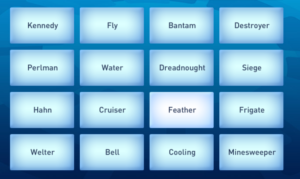For the first time anyone could remember, the Mathematical Ninja trembled with fear. He’d pulled his scary face, and it hadn’t worked: Victoria Coren Mitchell had simply said “you don’t scare me” and he had no idea what to do next.

“It’s time for the wall game,” she said.

“Right,” thought the Mathematical Ninja, in a bit of a fluster. “I can’t think straight… I’m going to have to guess, and guess fast.”

### Some background

In Only Connect, the Wall Game consists of 16 tiles, which can be arranged into four groups of four, like this:You have two and a half minutes to put them into the correct groups - but once you have two groups right, you only have three more chances to sort out the last two sets.

Trouble was, the Mathematical Ninja had absolutely no knowledge of anything apart from maths and weaponry - and only one of those was going to help him here.

### The power of randomness

Guess, guess and guess again, he thought: I have nimble fingers. What are my chances of getting the first one correctly? Well, let’s start with the top-left tile, because it must be in a group. It has three friends from the remaining 15, so there’s a $\frac 15$ chance of hitting one of those. Then I’d have two of the remaining 14, and one of the last 13 - and $\frac{1}{5} \times \frac{1}{7} \times \frac{1}{13}$, or $\frac{1}{455}$. At one guess a second, that would give about a $\frac {2}{7}$ chance of getting a set within 150 seconds.

How did he get that? He’d have a $p = \frac{454}{455}$ chance of being wrong; the probabiiity of him being wrong 150 times in a row is $p^{150} \simeq 0.719$, making a little more than $\frac{2}{7}$ for him getting a match.

He didn’t much like those odds - but how about if he lucked out and got the first one?

Then he’d pick one at random from the twelve he hadn’t found - then he’d have a probability of $\frac{3}{11} \times \frac{2}{10} \times {1}{9} = \frac{6}{990} = \frac{1}{165}$ of guessing correctly. Given 2:30, you’d have about a 40% chance of getting that right.

And how about after that? Given eight tiles to split into groups of four, it’s the same method: you’ve a $\frac 37$ chance of matching your first choice, then $\frac{2}{6}$ of matching with the third, and $\frac{1}{5}$ of getting the last one right - making $\frac{1}{35}$. However, you only have three guesses - so your chance of being wrong with all three is $0.917$ or so - about a one-in-twelve chance.